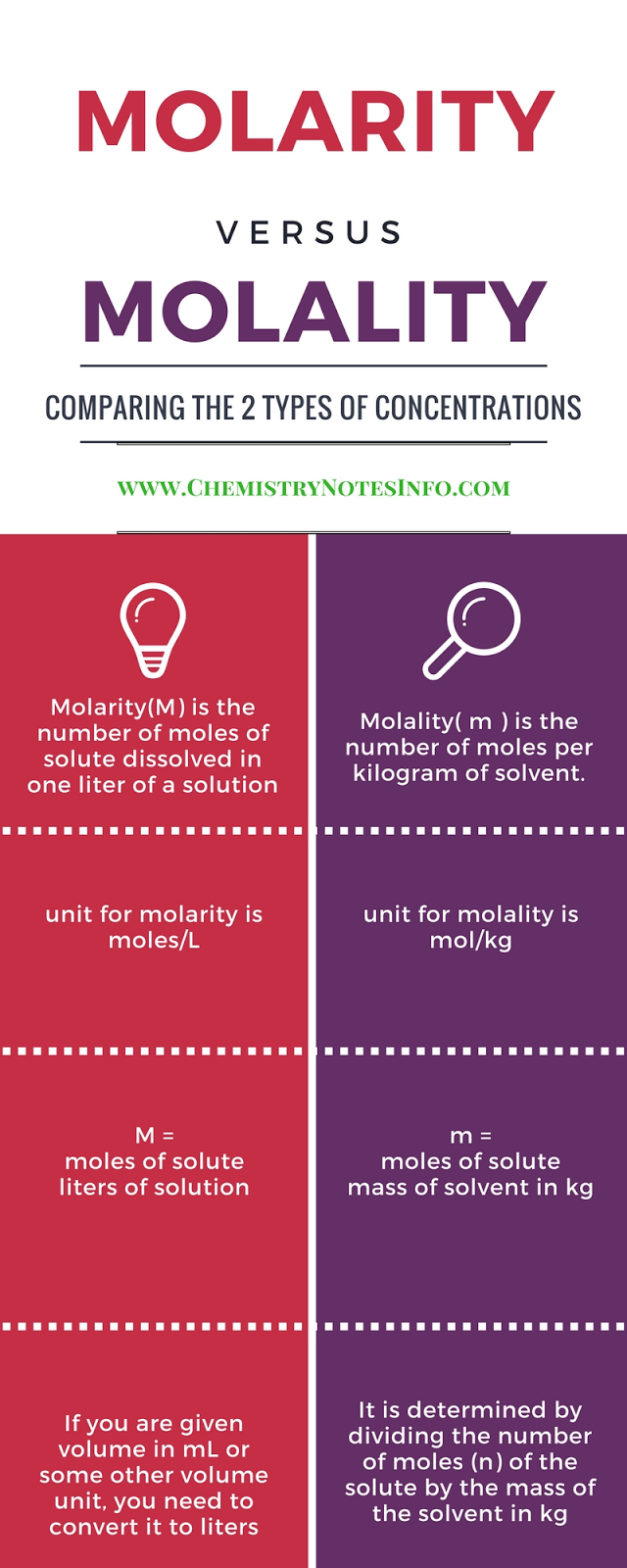# What is Molality?

It is the ratio of moles of substance to kilogram of solvent.
Where mole is weight in gram divided by molecular weight.
Molality is chemistry terminology.

## Molality (m) :-

No. of moles of the solute  Mass of solvent in Kilogram (Kg).

Unit =>   m = Moles/KgWhat is Molality